• SCIE, University of Electronic Science and Technology of China * 2.1.2单链表 四链表的特点 操作的顺序性 有平均次查找过程 离散存放 不受链表大小限制 不进行链点内容的搬移 查找操作数组效率优于链表 插入删除...
• C/C++ 编写函数，通过输入单向链表的头指针，对链表的value进行排序，返回排序后单向链表的头指针
• C语言 链表排序,源代码，代码清晰，适合初学者下载
• 主要介绍了JavaScript实现链表插入排序链表归并排序,较为详细的分析了插入排序和归并排序,对于学习JavaScript数据结构具有一定参考借鉴价值,需要的朋友可以参考下。
• 用双链表实现链表的合并以及链表的排序，其中包括链表的一些基本操作也有用于链表排序，链表合并的函数
• ## 链表排序

千次阅读 2019-06-04 22:24:09
在O(nlogn) 时间复杂度和常数级空间复杂度下，对链表进行排序。 示例 1: 输入: 4->2->1->3 输出: 1->2->3->4 示例 2: 输入: -1->5->3->4->0 输出: -1-&g...
1. 单链表排序 时间复杂度O(nlogn)----快速排序实现、归并排序实现
leetcode 148
在 O(n log n) 时间复杂度和常数级空间复杂度下，对链表进行排序。
示例 1:
输入: 4->2->1->3
输出: 1->2->3->4

示例 2:
输入: -1->5->3->4->0
输出: -1->0->3->4->5
代码实现
#include <iostream>
#include <queue>
using namespace std;

struct ListNode {
int val;
ListNode *next;
ListNode(int x) : val(x), next(NULL) {}
};

class Solution {
private:
// 快速排序实现
void qswap(int *a, int *b) {
int temp = *a;
*a = *b;
*b = temp;
}
ListNode* qsort(ListNode* nbegin, ListNode* nend) {
if (nbegin == nend || nbegin->next == nend)
return nbegin;
int value = nbegin->val;
ListNode* p = nbegin;
ListNode* q = nbegin;
while (q != nend) {
if (q->val < value) {
p = p->next;
qswap(&p->val, &q->val);
}
q = q->next;
}
qswap(&p->val, &nbegin->val);
return p;
}
void quicksort(ListNode* nbegin, ListNode* nend) {
if (nbegin == nend || nbegin->next == nend)
return;
ListNode* mid = qsort(nbegin, nend);
quicksort(nbegin, mid);
quicksort(mid->next, nend);
}

//归并排序实现
}

ListNode* dummyNode = new ListNode(-1);
ListNode* pre = dummyNode;
}
else {
}
pre = pre->next;
}

ListNode* resNode = dummyNode->next;
delete dummyNode;
return resNode;
}

return NULL;

while (cur2->next != NULL && cur2->next->next != NULL) {
pre = cur1;
cur1 = cur1->next;
cur2 = cur2->next->next;
}
if (cur2->next != NULL) {
pre = cur1;
cur1 = cur1->next;
}
pre->next = NULL;
return cur1;
}

public:
}
// 使用快速排序实现

// 使用归并排序实现
}
};
参考博客：单链表排序----快排 & 归并排序
展开全文• 文章目录链表排序与数组排序的区别借助外部空间冒泡排序插入排序归并排序快速排序 链表排序与数组排序的区别 数组的排序几乎所有人都很熟悉了，常用的算法插入、冒泡、归并以及快排等都会或多或少依赖于数组可以在O...


文章目录
链表排序与数组排序的区别借助外部空间冒泡排序插入排序归并排序快速排序

链表排序与数组排序的区别
数组的排序几乎所有人都很熟悉了，常用的算法插入、冒泡、归并以及快排等都会或多或少依赖于数组可以在O(1)时间随机访问的特点。
链表排序一般指单链表排序，链表是不支持随机访问的，需要访问后面的节点只能从表头顺序遍历，所以链表的排序是一个相对比较复杂的问题。
那么怎样进行链表排序呢？
借助外部空间
既然数组排序简单，那可以借助数组进行排序：
把链表中的值一次遍历导入数组（时间复杂度O(n)）对数组进行排序（可以选择各种算法，假设选择快排，时间复杂度O(nlogn)）把排序后的元素依次放入链表的节点内，也是一次遍历
综上，该方法时间复杂度为O(nlogn)，空间复杂度主要为额外的数组空间(如果选择其他排序算法，可能还需要更多的空间)O(n)。
不过这种方法有点投机取巧的意思：
class Solution {
public:
vector<int> a;
while(p != NULL){
a.push_back(p->val);
p = p->next;
}
delete p;
sort(a.begin(), a.end());	//可以自己写个快排，会提升不少效率
for(const auto &c : a){
q->val = c;
q = q->next;
}
delete q;

}
};

冒泡排序
链表可以使用冒泡排序吗？
我们知道，冒泡排序一次冒泡会让至少一个元素移动到它应该处于的位置，n次（可能小于n次）冒泡之后那就所有元素都在自己应该在的位置了。
对于节点pre，cur，如果两者不满足大小关系要求，在数组排序里面，我们会交换两者的值，但是在链表排序里面，有两种选择：
交换节点的值，更简单，但是很多题目会要求不能单纯的交换节点的值(⊙﹏⊙)交换节点，复杂，主要体现在两个节点的前驱后继的处理上（因为只能单向遍历）。
第一种情况，可以交换节点的值：
class Solution {
public:
for(int i = 0; i < n; ++i){
bool flag = 0;
for(int j = 0; j < n-i-1; ++j){
if(cur != NULL && pre->val > cur->val){
swap(pre->val, cur->val);
flag = 1;
}
pre = pre->next;
cur = cur->next;
}
if(!flag)   break;
}
}
int n = 0; // 记录节点个数
while(p != NULL){
++n;
p = p->next;
}
}
};

以上代码时间复杂度为O(

n

2

n^2

)， 空间复杂度O(1)。
可以交换节点值的时候代码就会清晰明了，只需要处理好边界问题即可。不过上述代码表现不太好的地方是时间复杂度为O(

n

2

n^2

)，比如在148. 排序链表 里面，就会因为超出时间限制而没法通过最后一个测试用例。
可以看到，如果可以交换节点的值，那使用插入、快排这些顺序遍历可以实现的算法都是可以的（当然，快排就不能使用双指针法了）。
第二种情况，不能交换节点的值： 前面说过，交换节点的难点在于前驱后继的处理，如果当前要交换的pre和cur如下所示：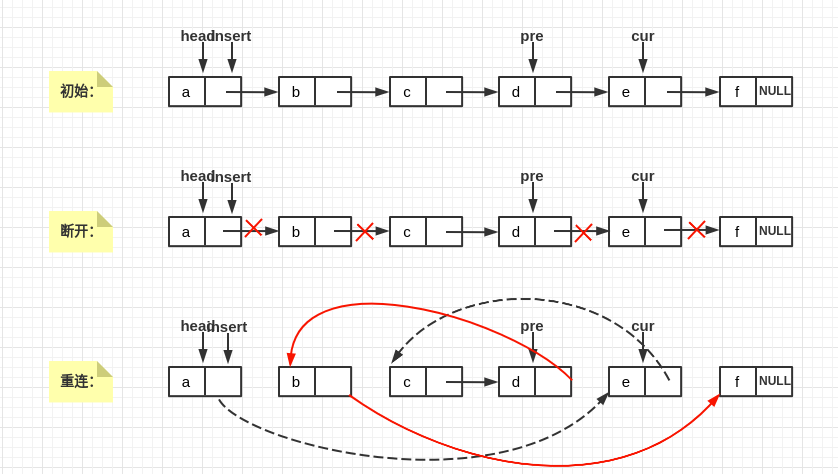可以看到要交换节点，我们需要：
随时记录pre,cur的前驱两个临时变量保存pre,cur的后继更改指针
看上去就头很大。但事实上链表排序完全没必要使用冒泡，因为对于链表来说，插入排序比冒泡排序更容易理解也更简单，具体可以看下一部分插入排序的讲解。这儿就不贴冒泡的代码了（其实是因为没写(⊙﹏⊙)）。
插入排序
数组的插入排序，简单来说就是每次在未排序区间取元素，然后将该元素插入到已排序空间的合适位置，直到未排序空间为空。
链表的插入排序处理逻辑与数组的是一致的。
插入，顾名思义就是在两个节点之间插入另一个节点，并且保持序列是有序的。上一节为什么说插入比冒泡更简单呢（无论是链表还是数组，一般都优先使用插入排序），看下面的图，如果当前要将节点cur插入到节点pre之后：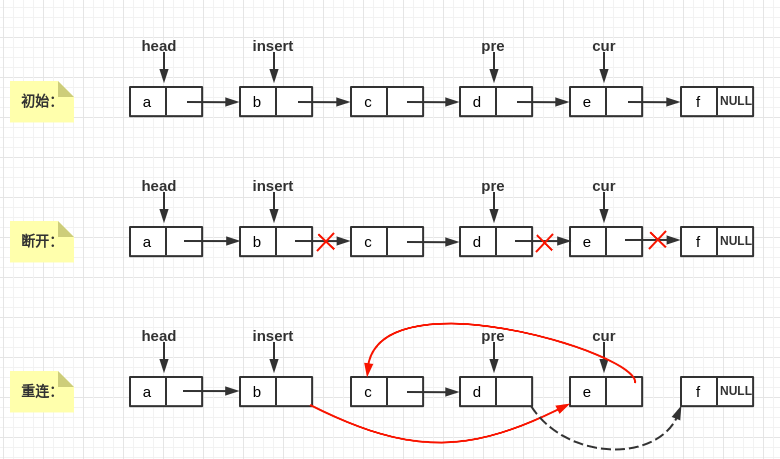可以看到整体操作逻辑简单了许多：我们只需要知道cur的前驱和插入位置（其实就是要插在哪个节点之后）就可以啦。
链表的插入排序对应：
147. 对链表进行插入排序 - 力扣（LeetCode） LeetCode第 147 题：对链表进行插入排序(C++)
贴一个代码：
class Solution {
public:
auto dummy = new ListNode(-1);
auto pre = head; // 当前节点的前驱
while(cur != NULL){
auto tmp = dummy;
if(pre->val >= cur->val){ //需要进行插入
while(tmp->next->val < cur->val) //从第一个节点开始寻找插入位置
tmp = tmp->next; // cur应该插入在tmp后面
pre->next = cur->next; //断开节点cur
cur->next = tmp->next; //插入
tmp->next = cur;
cur = pre->next; //继续处理下一个节点
}
else{ //无需插入
pre = pre->next;
cur = cur->next;
}
}
return dummy->next;
}
};

时间复杂度为O(

n

2

n^2

)，空间复杂度为O(1)。
可以进行优化：因为上述代码我们每次的插入点都是从头开始查找的，但是其实可以先和上一个插入点tmp进行比较，如果当前节点值更大的话，查找插入点的起始点就可以是tmp节点，否则再从头开始查找插入点。
class Solution {
public:
auto dummy = new ListNode(-1);
auto pre = head; // 当前节点的前驱
auto tmp = dummy; //tmp用于记录上一次的插入位置
while(cur != NULL){
if(cur->val < tmp->val) tmp = dummy; //如果当前值比上一次插入点的值要小，就只能从头查找插入点,否则，插入点的查找可以从上一个插入点之后开始查找
if(pre->val >= cur->val){ //需要进行插入
//tmp开始寻找插入位置
while(tmp->next->val < cur->val)    tmp = tmp->next; // cur应该插入在tmp后面
pre->next = cur->next; //断开节点cur
cur->next = tmp->next; //插入
tmp->next = cur;
cur = pre->next; //继续处理下一个节点
}
else{ //无需插入
pre = pre->next;
cur = cur->next;
}
}
return dummy->next;
}
};

归并排序
后面的归并排序和快速排序时间复杂度都是O(nlogn)，空间复杂度则略有不同，两者的思想都是分治加递归（递归不是必要的），只不过一个自顶向下，一个自下而上。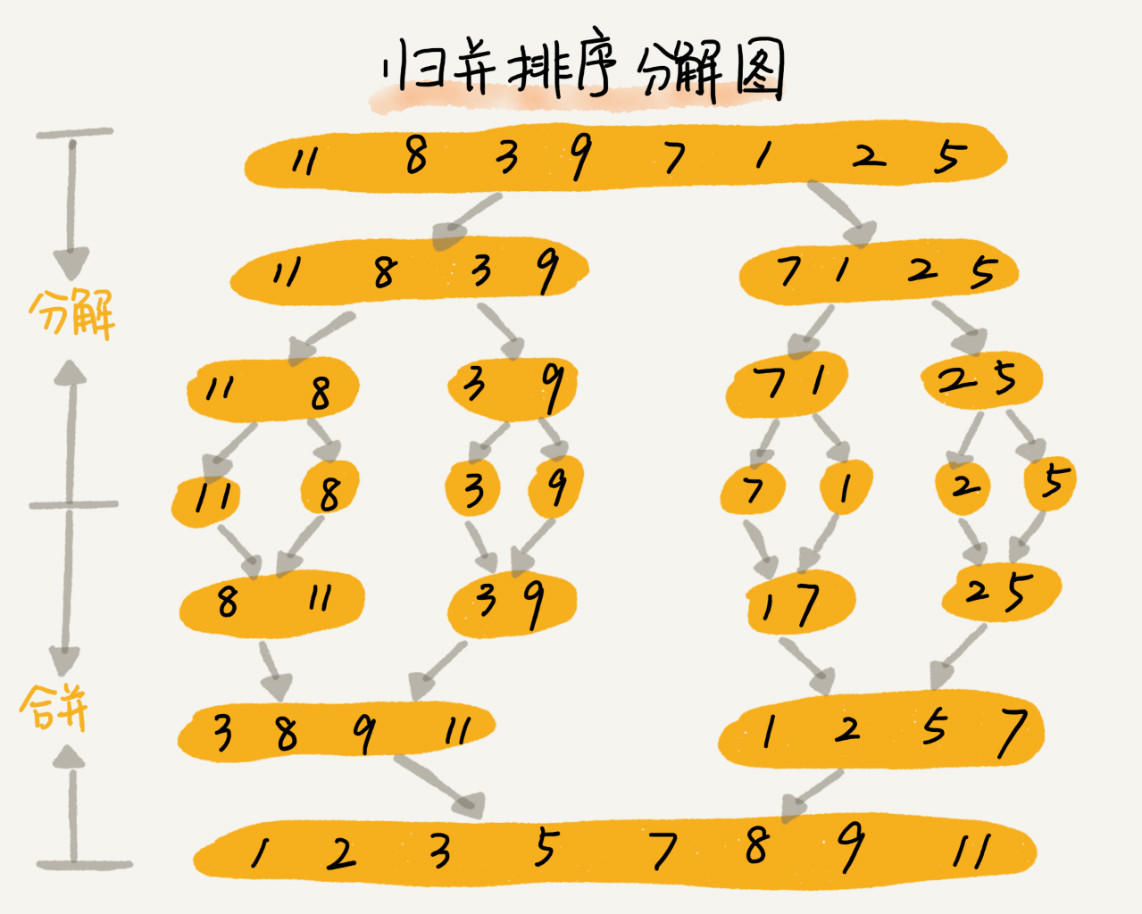先来看归并：
归并排序其实是链表排序的主流方法。 归并主要分为分割和合并两大部分，入栈的时候分割，出栈的时候合并，这也是归并常常采用递归实现的原因。
首先，如何分割？数组里面我们是这样操作的：
void merge_sort_c(vector<int> &a, int beg, int end){
if (beg >= end) return;
auto mid = beg +(end-beg) / 2;
merge_sort_c(a, beg, mid);
merge_sort_c(a, mid+1, end);
merge(a, beg, mid, end);
}

主要就是获取中间位置，然后分治，但是对于链表来说，怎样寻找中间节点呢？
直接法：第一次遍历获取节点个数，第二次遍历就可以定位到中间节点了快慢指针法：慢指针一次走一步，快指针一次走两步，当快指针达到末尾的时候，慢指针正好会指向中间节点，只用一次遍历。
此处就是用快慢指针法来定位中间节点，从而达到分割的目的：
ListNode *slow = head, *fast = head->next;
// fast一次要走两步
while(fast != NULL && fast->next != NULL){
slow = slow->next;
fast = fast->next->next;
}

auto tmp = slow->next;//右边链表的开头
slow->next = NULL; //切断形成左半边链表

其实就是将链表切成两部分，也就是分治嘛。。。
那么又如何处理合并呢？ 数组的merge函数我们已经很熟悉了：
需要一个辅助数组，大小与A[p…r]相同，双指针i,j分别指向两个待合并数组开头，假设为A[p…q]，A[q+1,r]：
比较A[i]与A[j]，将较小者放入辅助数组并移动指针指向下一个元素…直到有一个数组处理完成然后再将未处理完成的数组剩余元素一次性放入辅助数组（此时辅助数组里就是排序好的数组了）最后将辅助数组里的元素搬运回原数组
可以看到处理的空间复杂度为O(n)，主要是辅助数组的开销。那这儿为什么要用辅助数组呢？主要是因为如果不使用辅助数组的话，就只能进行元素插入，而数组存储空间是连续的，意味着插入操作将会带来大量的元素移动（O(n)时间复杂度），这是接受不了的。
void merge(vector<int> &a, int beg, int mid, int end){
vector<int> tmp(end-beg+1, 0);
int i = beg, j = mid + 1;
int k = 0;
while (i <= mid && j <= end){
if (a[i] <= a[j])
tmp[k++] = a[i++];
else
tmp[k++] = a[j++];
}
// 处理剩余部分
int start = (i == mid + 1) ? j : i;
int tail = (i == mid + 1) ? end : mid;
while (start <= tail)
tmp[k++] = a[start++];

for (const auto &c : tmp)	a[beg++] = c;
}

但是，如果处理链表就不存在这个问题，因为链表不是连续存储的，插入删除的时间复杂度都是O(1)。 所以链表的合并并不需要O(n)的空间复杂度，因为我们不需要类似辅助数组这样的东西，我们仅仅是调整一下相应的指针就能达到合并的目的。
void merge(ListNode *left, ListNode *right, ListNode *h){
while(left != NULL && right != NULL){
if(left->val < right->val){
h->next = left;
left = left->next;
}else{
h->next = right;
right = right->next;
}
h = h->next; //随时更新h,好在h后面加入新节点
}
h->next = left ? left : right; //处理剩余部分
}

那上述merge的空间复杂度是O(1)还是O(logn)呢？其实我也不太确定，因为递归的函数栈确实是logn,这个算不算空间复杂度也是众说纷纭。
递归实现的链表归并排序代码：
class Solution {
public:
ListNode* merge(ListNode *left, ListNode *right){
while(left && right){
if(left->val < right->val){
h->next = left;
left = left->next;
}else{
h->next = right;
right = right->next;
}
h = h->next;
}
h->next = left == NULL ? right : left;
}
while(fast && fast->next){
slow = slow->next;
fast = fast->next->next;
}
slow->next = NULL;//左边切断开
return merge(left, right);
}
}
};

可以看一下这个题：
148. 排序链表 - 力扣（LeetCode）
题目要求常数级的空间复杂度，如果递归栈算在空间复杂度里面的话，那上述递归的归并排序代码就不符合要求了。那不符合要求并不代表归并排序不行，因为递归只是算法的特定实现方式而已，我们也可以使用迭代来实现链表的归并排序。
看下面的示意图：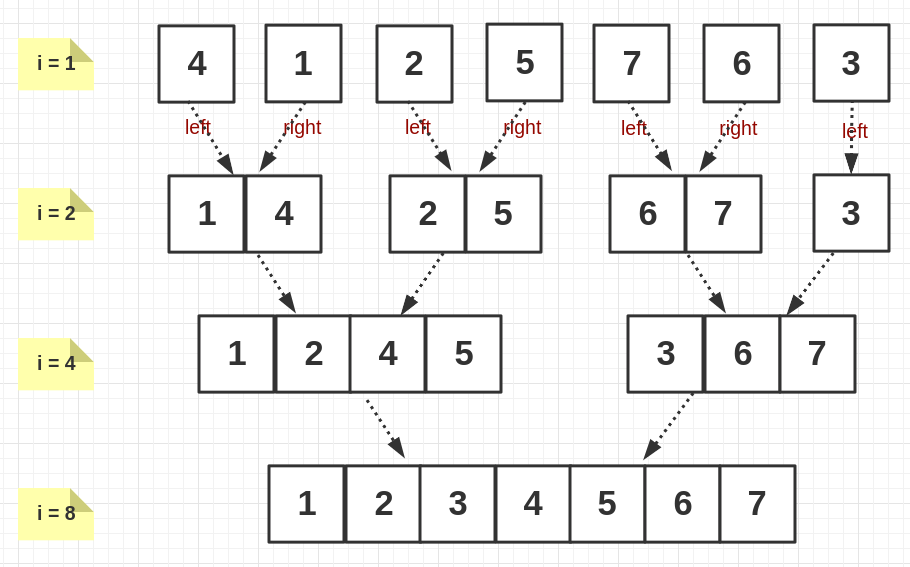第一轮迭代 1 + 1-> 2，第二轮迭代 2 + 2-> 4，以此类推，总的迭代次数应该为logn，即迭代变量i每次都会变为之前的两倍，i即为每次迭代需要合并的块含有的元素数量。
首先，最外层迭代次数为logn内层迭代变量j递增方式为：j += 2*i，每次合并两块，合并完两块之后下一次循环合并再后面的两块…
迭代实现的代码会长了很多（很长。），但其实逻辑更简单，具体看注释吧：
class Solution {
public:
int n = 0;
for(auto i = head; i != NULL; i = i->next)  ++n; //n为链表长度
auto dummy = new ListNode(-1);
// 总体循环次数为logn
for(int i = 1; i < n; i += i){
auto beg = dummy;
// 最开始1个和1个合并为两个，然后2,2->4, 4,4->8
for(int j = 0; j + i < n; j += 2*i){ //j += 2*i为下一块的起点
auto left = beg->next;
auto right = left;
for(int k = 0; k < i; ++k)  right = right->next;//right指向第二块的起点,每块有i个节点，越过i个节点即可
// merge第一块和第二块，起点分别为left, right
// 第一块的节点数为i, 第二块的节点数可能小于i（为i-1）,因为节点个数有奇偶之分,所以需要检查right != NULL
int p = 0, q = 0;//计数
while(p < i && q < i && right != NULL){
if(left->val <= right->val){
beg->next = left;
left = left->next;
++p;
}else{
beg->next = right;
right = right->next;
++q;
}
beg = beg->next;
}
while(p < i){// 可能还有剩余未处理的
beg->next = left;
beg = beg->next;
left = left->next;
++p;
}
while(q < i && right != NULL){
beg->next = right;
beg = beg->next;
right = right->next; //right会指向下一块的起点
++q;
}
// 处理完之后beg指向的是两块中（已经排序好）元素最大的那个节点
beg->next = right; //调整beg位置，将beg和下一块连接起来
}
}
return dummy->next;
}
};

迭代实现就满足了上面的题目时间复杂度O(nlogn)，以及常数级的空间复杂度的要求。
快速排序
终于到快速排序了╮（﹀_﹀）╭
数组快排简单说就是找分区点pivot(第一个或者最后一个)，然后将小于pivot的元素全部放到它的左边，大于它的元素则放到右边，自上而下，分治+递归。
递归过程简单明了：
void quickSort(vector<int> &a, int beg, int end){
if (beg >= end)	return;
auto q = partition(a, beg, end); //获取分区结点
quickSort(a, beg, q-1);
quickSort(a, q+1, end);
}

重点在于partition函数的实现，快速排序的多种实现方式_，贴两个常用的：
双指针法：
// 双指针(pivot为末尾)
int partition1_1(vector<int> &a, int low, int high){
auto pivot = a[high];
int i = low, j = high;
while (i < j){
// 先看左边(顺序不能错)
while (i < j && a[i] <= pivot)	++i;
// 再看右边
while (i < j && a[j] >= pivot)	--j;
swap(a[i], a[j]);
}
swap(a[high], a[i]);
return i;
}

以及这个不知道名字的方法：
// 单边法(末尾为pivot)
int partition2_1(vector<int> &a, int low, int high){
auto pivot = a[high];
int i = low, j = low;//i指向以处理区间右边界，j用来遍历元素
while (j < high){
if (a[j] < pivot)
swap(a[i++], a[j]); //已处理区间多了一个元素，右边界增加1
++j;
}
swap(a[high], a[i]);
return i;
}

如果以开头作为pivot:
// 单边法(开头为pivot)
int partition2_2(vector<int> &a, int low, int high){
auto pivot = a[low];
int i = low+1, j = low+1;//i指向以处理区间右边界，j用来遍历元素
while (j <= high){
if (a[j] < pivot)
swap(a[i++], a[j]);//已处理区间多了一个元素，右边界增加1
++j;
}
swap(a[low], a[i-1]);
return i-1;
}

为什么要强调第二种我叫不上名字的方法以及它的以开头为pivot的版本呢，因为接下来我们要用在链表的快速排序上面。
首先想想我们为什么不用双指针法，因为双指针需要从后往前遍历啊，而单链表是没法从后往前遍历的。
所以我们需要第二种方法，因为它一直在从前往后遍历，而且最好是以链表开头作为pivot，因为也没法以链表结尾作为pivot(需要遍历到末尾)。那么现在我们就可以对链表进行partition了。
注意，这儿的partition是以排序可以交换节点的值为前提的。
partition函数逻辑和上一个处理数组的逻辑是一样的，细节上略有差别，主要因为：
处理数组的，可以访问到a[i-1],也就是边界的前一个元素而链表则无法访问到上一个节点
class Solution {
public:
while(cur != tail){
if(cur->val < pivot){
left = left->next;
swap(left->val, cur->val);
}
cur = cur->next;
}
return left;
}
quickSort(q->next, tail);
}
}
};

既然上面的快排代码是建立在可以交换节点值的前提上的，那么如果不可以交换节点的值，只能交换节点，应该怎么写呢？
我们要做的依然是partition操作， 还是使用头结点作为pivot，把链表分为3部分:pivot左边，pivot，pivot右边。
具体的还是看注释吧，重点还是在划分部分：
class Solution {
public:
auto left = new ListNode(-1); //左边部分的开头
auto right = new ListNode(-1); //右边部分的开头
ListNode *l = left,*r=  right;
for(auto cur = head; cur != NULL; cur = cur->next){
if(cur->val < pivot){
l->next = cur;
l = l->next;
}else{
r = r->next;
}
}
l->next = NULL;
r->next = NULL;
// 现在left->next即为左边部分的头结点
//right->next即为pivot
//riight->next->next即为右边部分的头结点
auto p = quicksort(left->next);
auto q = quicksort(right->next->next);
//上面分治的时候切断了， 最后需要再进行连接
//p可能为NULL
if(!p)   left->next = right->next;
else{
left->next = p;
while(p->next != NULL)  p = p->next; //p指向末尾
p->next = right->next;
}
right->next->next = q;
return left->next;
}
}
};

换个写法：
class Solution {
public:
auto left = new ListNode(0);
auto right = new ListNode(0);
ListNode *l = left, *r = right;
for(auto cur = head->next; cur != NULL; cur = cur->next){
if(cur->val < pivot){
l->next = cur;
l = l->next;
}else{
r->next = cur;
r = r->next;
}
}
l->next = NULL;//断开形成左边链表
r->next = NULL;//断开形成右边链表
auto p = quicksort(left->next);
auto q = quicksort(right->next);
//最后再进行连接
//p可能为NULL
else{
left->next = p;
while(p->next != NULL)  p = p->next;
}
return left->next;
}
}
};

展开全文• 经典的双向链表排序算法。涵盖创建，删除，排序，获取，增加等
• 对链表进行插入排序，是最简单的一种链表排序算法，用于插入排序是迭代的，所以每次只移动一个元素，直到所有元素可以形成一个有序的输出列表。        每次迭代中，插入排序只从输入数据中移...
插入排序
对链表进行插入排序，是最简单的一种链表排序算法，用于插入排序是迭代的，所以每次只移动一个元素，直到所有元素可以形成一个有序的输出列表。        每次迭代中，插入排序只从输入数据中移除一个待排序的元素，找到它在序列中适当的位置，并将其插入。重复直到所有输入数据插入完为止。        插入排序的时间复杂度为O(N^2)，空间复杂度为O（1）
class Solution {
while(move!=null&&move.next!=null){
move=move.next;
}
}
ListNode temp=node.next;
node.next=temp.next;
return true;
}
}
temp.next=node.next;
node.next=temp;
return false;
}
}

归并排序
对于归并排序排序在数组排序中的运用，详细请点击此处。这里主要介绍归并排序在链表排序中的运用。        在使用归并排序算法进行链表排序时，其基本思想是将链表细分成一个个子链表，将子链表进行排序，然后再将相邻的两个有序子链表进行合并，得到更长的有序链表，最后一步步得到整个有序链表，子链表进行合并排序时需要用到合并两个有序链表算法。        归并链表排序的实现方式一共有两种，递归实现和非递归实现，两种实现方式的时间复杂度都是O(nlogn），但是由于递归实现调用函数时需要消耗大量栈空间，所以递归调用的空间复杂度是O（logn）。对于非递归调用，空间复杂度就是O（1）。
递归代码：
class Solution {
}
while(fast!=null&&fast.next!=null){
fast=fast.next.next;
if(fast!=null)
slow=slow.next;
}
slow.next=null;
}
}else{
}
move=move.next;
}
}
}


非递归代码：
class Solution {
return null;
int length=0;
while(end!=null){
length++;
end=end.next;
}
for(int i=1;i<length;i<<=1){
while(cur!=null) {
ListNode start1 =cur;
for (int j = 1; j < i&&cur.next!=null; j++) {
cur = cur.next;
}
ListNode start2 = cur.next;
cur.next = null;
cur = start2;
for (int j = 1; j < i && cur != null&&cur.next!=null; j++) {
cur = cur.next;
}
if(cur!=null){
ListNode temp=cur;
cur=cur.next;
temp.next=null;
}
prev.next = mergeList(start1, start2);
while(prev.next!=null){
prev=prev.next;
}
}
}
}
}else{
}
move=move.next;
}
}
}

展开全文算法 java
• 链表归并排序的过程如下。 找到链表的中点，以中点为分界，将链表拆分成两个子链表。寻找链表的中点可以使用快慢指针的做法，快指针每次移动 2 步，慢指针每次移动 1步，当快指针到达链表末尾时，慢指针指向的链表...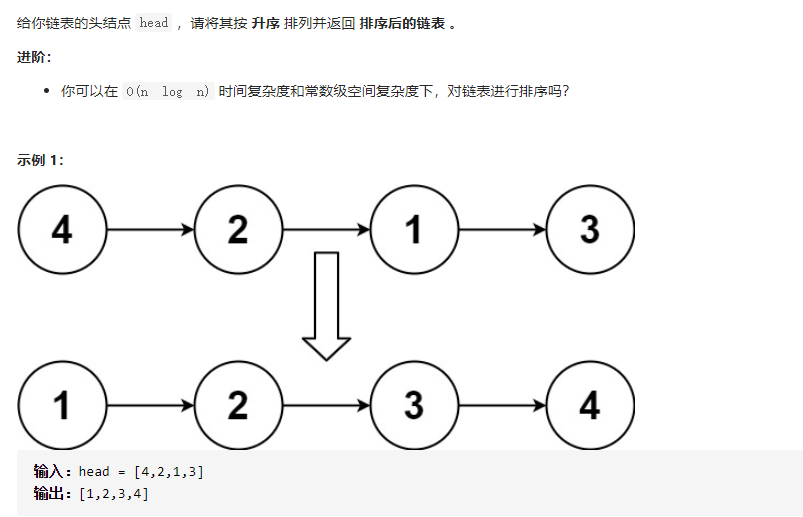我们可以试用归并排序解决： 对链表归并排序的过程如下。
找到链表的中点，以中点为分界，将链表拆分成两个子链表。寻找链表的中点可以使用快慢指针的做法，快指针每次移动 2 步，慢指针每次移动 1步，当快指针到达链表末尾时，慢指针指向的链表节点即为链表的中点。
对两个子链表分别排序。
将两个排序后的子链表合并，得到完整的排序后的链表
上述过程可以通过递归实现。递归的终止条件是链表的节点个数小于或等于 1，即当链表为空或者链表只包含 1 个节点时，不需要对链表进行拆分和排序。

class Solution {
public:
}

ListNode* mergesort(ListNode* head, ListNode* tail) {
}
}
while (fast != tail) {
slow = slow->next;
fast = fast->next;
if (fast != tail) {
fast = fast->next;
}
}

return merge( mergesort(head, slow),  mergesort(slow, tail));
}

while (temp1 != nullptr && temp2 != nullptr) {
if (temp1->val <= temp2->val) {
temp->next = temp1;
temp1 = temp1->next;
}
else {
temp->next = temp2;
temp2 = temp2->next;
}
temp = temp->next;
}
if (temp1 != nullptr) {
temp->next = temp1;
}
else if (temp2 != nullptr) {
temp->next = temp2;
}
}
};

快速排序不能随机选取节点，时间复杂度太高所以会超时
class Solution {
public static ListNode sortList(ListNode head) {
}

public static ListNode quickSort(ListNode head ,ListNode end){
while (p != end){
ListNode next = p.next;
}
else { //尾插
utail.next = p;
utail = p;
}
p = next;
}
utail.next = end;
return node;
}
}


展开全文链表 指针 归并排序
• ## 链表排序算法

万次阅读 多人点赞 2018-04-18 10:34:09
排序算法概述盗个图转自：https://www.cnblogs.com/onepixel/articles/7674659.html排序算法复杂度由于是链表排序，首先定义链表节点数据结构common.htypedef struct Node LNode; struct Node { int data; LNode ...插入排序 归并排序
• ## C语言-链表排序

千次阅读 2019-05-13 20:00:54
C语言-链表排序 题目描述 已有a、b两个链表，每个链表中的结点包括学号、成绩。要求把两个链表合并，按学号升序排列。 输入 第一行，a、b两个链表元素的数量N、M,用空格隔开。 接下来N行是a的数据 然后M行是b的...
• 链表排序相对数组的排序更为复杂些，也是考察求职者是否真正理解了排序算法（而不是“死记硬背”） 链表的插入排序 public class LinkedInsertSort { static class ListNode { int val; ListNode next; ...
• ## c++ 链表排序

千次阅读 2019-05-11 19:08:50
node *bubbleSortList(node *head) { if (head == NULL || head->next == NULL) return head; node *p = NULL; bool isChange = true; while (p != head->next->next && i...算法
• ## 双向链表排序

万次阅读 2018-07-17 20:52:18
•算法 java 归并
• 在 O(n log n) 时间复杂度和常数级空间复杂度下，对链表进行排序。 示例 1: 输入: 4-&gt;2-&gt;1-&gt;3 输出: 1-&gt;2-&gt;3-&gt;4 示例 2: 输入: -1-&gt;5-&gt;3-&...快速排序 算法快速排序 java
• C++双向链表排序 建立一个长度为n的带头结点的双向链表，使得该链表中的数据元素递增有序排列。（必须使用双向链表完成，数据类型为整型。） 输入 第一行：双向表的长度； 第二行：链表中的数据元素。 输出 ...C 数据结构
• 链表排序 1、要求：  Sort a linked list in O(n log n) time using constant space complexity. 2、代码： /** * Definition for singly-linked list. * struct ListNode { * int val; * ListNode *ne归并排序
• 链表冒泡排序 比较两个相邻的元素大小，每一趟会把较大（或较小）的数放在往后移。链表冒泡排序思想：设置两个指针，一个是当前指针，一个是尾指针，当前的指针指向头节点，将尾指针赋为空，当当前的指针不等于尾...
• //输入一个数列以0为结束标志，建立链式线性表，查找其中最大的数并输出删除释放节点，然后对剩余的进行排序，并输出释放节点。
• 1、演示链表排序的算法流程； 2、讲解预定义语句的作用；linklist
• 使用c++的类和结构体指针，对链表进行排序，而且排序链表和排好序的链表是同一个链表，不借助其他临时链表...